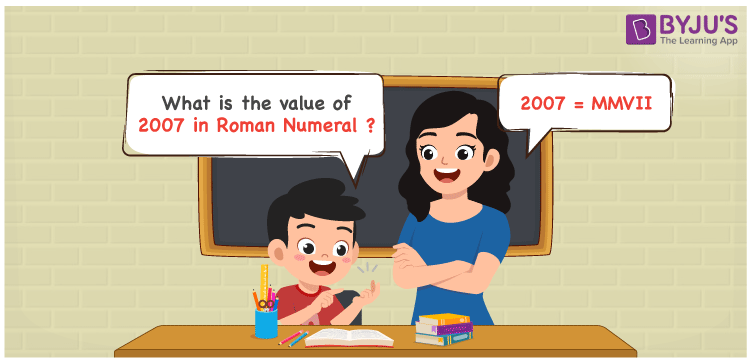# 2007 in Roman Numerals

2007 in Roman Numerals is given by MMVII. The symbolic representation of numbers that do not follow a place value system is known as Roman Numerals. Learn the easy method of converting Roman Numerals into numbers by downloading the Roman Numerals Conversion in PDF format free. In this article, we will learn how to write the number 2007 in Roman Numerals along with FAQs with a complete explanation.

 Number Roman Numeral 2007 MMVII

## How to Write 2007 in Roman Numerals?The method used to express the number 2007 in Roman Numerals is shown below

• Break the number 2007 into the simplest form
• 2007 = 1000 + 1000 + 5 + 1 + 1
• Write their appropriate Roman Numerals and add/subtract them
• 2007 = M + M + V + I + I = MMVII
• Hence the value of 2007 in Roman Numerals is MMVII

## Video Lesson on Roman Numerals## Frequently Asked Questions on 2007 in Roman Numerals

Q1

### What is 2007 in Roman Numerals?

2007 in Roman Numerals is MMVII.
Q2

### What number should be added to 1000 to get 2007? Express the answer in Roman Numerals.

2007 – 1000 = 1007. Hence the number 1007 should be added to 1000 to get 2007. The number 1007 in Roman Numerals is MVII. Thus 1007 in Roman Numerals is MVII.
Q3

### What is the difference between 2050 – 2007 in Roman Numerals?

2050 – 2007 = 43. The number 43 in Roman Numerals is written as XLIII. Thus the difference between 2050 – 2007 in Roman Numerals is XLIII.